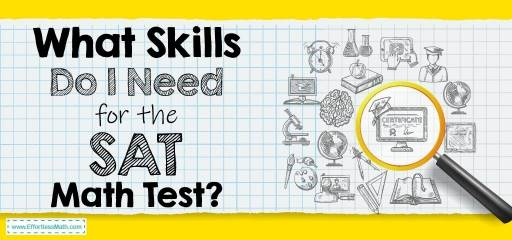# What Skills Do I Need for the SAT Math Test?The SAT is a critical component used by colleges to assess how well you will do academically with them, especially when combined with your high school GPA.

Math is a significant component of the SAT, and you may then be wondering, what skills do I need for the SAT math test to achieve the best results possible?

First off, the SAT math test is not just about knowing how to solve an equation. Instead, the test assesses how well you understand the topics you would need to use most commonly during college and work life.

You may find some questions are multi-step because single calculations rarely solve anything outside of tests, and a single scenario will be used for multiple questions.

The Absolute Best Book to Ace the SAT Math Test

## SAT Math Test Format

The SAT math test is broken into 2 sections, calculator allowed and calculator not allowed.

The majority of the questions will be multichoice; however, you will find some grid-in questions, which require you to provide an answer rather than just selecting one from a list.

The SAT math test with a calculator is not allowed is 25 minutes and 20 questions long, 15 multichoice questions, and 5 grid-in.

The SAT math test with a calculator allowed is 55 minutes and 38 questions long, 30 multichoice questions, and 8 grid-in.

## SAT Math Test Sections

The SAT math test is broken into 3 main sections with a supplemental section at the end.

### Heart of Algebra

• Understand how to turn word problems into expressions, equations, or inequalities.
• Be able to calculate linear equations or inequalities or a system of, with 1 or 2 variables.
• Understand linear equations, expressions, or inequalities in context.
• Comprehend how linear graphs relate to an equation or system of equations or inequalities.

### Problem-Solving and Data Analysis

• Be able to use ratios, rates, and proportions to solve problems.
• Be able to use percentages to solve problems.
• Unit conversions.
• Be able to predict a line or curve of best fit for a scatterplot.
• Comprehend the difference between linear and exponential growth.
• Calculate probabilities from provided data.
• Understand how to calculate mean, mode, median, and range of data, and comprehend how these, along with standard deviation, affect the shape, center, and spread of data.
• Make and explain statistical inferences from data.

• Understand how to use a quadratic or exponential function to model real-life contexts.
• Be able to simplify expressions with exponents.
• Understand nonlinear expression in its context.
• Be able to isolate variables in nonlinear equations.
• Be able to solve quadratic equations.
• Be able to perform operations: addition, subtraction, multiplication, and division, with polynomial expressions.
• Understand equations containing variables in the denominator.
• Be able to solve systems of equations (quadratic and linear equations).
• Understand how the zeros and factors of a polynomial relate to one another and the polynomial’s graph.
• Understand how a nonlinear graph relates to an equation or system of nonlinear equations.
• Comprehend function notation and how transformations/compositions are performed in this notation.

• Use volume and area formulas, which will be provided.
• Understand how to calculate missing information when a transversal cuts parallel lines or when similar or congruent triangles are provided.
• Understand how to calculate right triangles using the Pythagorean theorem or trigonometric ratios.
• Understand the relationship between similar triangles, trigonometric ratios, and right triangles.
• Understand how to calculate missing values in a circle.
• An ability to convert between radian and degree measures.
• Understand the standard form for the equation of a circle in the coordinate plane.
• Understand operations between complex numbers.

Best SAT Math Prep Resource

## What the SAT Math test is measuring

The SAT math test is not just measuring that you can answer 1 math problem. Instead, it’s assessing your ability to use your math knowledge to solve a series of equations that would be presented in college or the real world.

You need to understand the theories taught to you and then apply them to the questions being asked. Some of the questions will allow you to guess from the multichoice options; however, others will need you to come up with the answer and provide it in the grid-in spaces provided.

Both the calculator-allowed and calculator not allowed portions of the test are also essential to assess your knowledge. Can you use a calculator efficiently to solve problems when needed?

The calculator is only as good as the user’s and isn’t a magical tool that will provide answers for you.

You’re also assessed in calculating and solving problems when a calculator is unavailable to you, which is sometimes the case when working on problems outside of school.

## Final Thoughts

The SAT math test assesses your understanding of the math taught over your high school years, so thorough knowledge is required. While you can study and prep for specific test areas, your comprehension of these topics should have started long ago.

You can study by yourself or with a group, you can enroll in a specific SAT math study class, or can buy a self-paced study guide to assist you.

Whatever you do, make sure you take practice tests and find areas to focus on to get the best result possible.

## College Entrance Tests

Looking for the best resource to help you succeed on the SAT Math test?

The Best Books to Ace the SAT Math Test

### What people say about "What Skills Do I Need for the SAT Math Test? - Effortless Math: We Help Students Learn to LOVE Mathematics"?

No one replied yet.

X
49% OFF

Limited time only!

Save Over 49%

SAVE \$34

It was \$69.99 now it is \$35.99